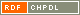# Show document

Title: Almost fully optimized infinite classes of Boolean functions resistant to (fast) algebraic cryptanalysis Pašalić, Enes (Author)http://dx.doi.org/10.1007/978-3-642-00730-9_25 English Not categorized 1.08 - Published Scientific Conference Contribution FAMNIT - Faculty of Mathematics, Science and Information Technologies In this paper the possibilities of an iterative concatenation method towards construction of Boolean functions resistant to algebraic cryptanalysis are investigated. The notion of ▫$\mathcal{AAR}$▫ (Algebraic Attack Resistant) function is introduced as a unified measure of protection against classical algebraic attacks as well as fast algebraic attacks. Then, it is shown that functions that posses the highest resistance to fast algebraic attacks are necessarily of maximum ▫$\mathcal{AI}$▫ (Algebraic Immunity), the notion defined as a minimum degree of functions that annihilate either ▫$f$▫ or ▫$1+f$▫. More precisely, if for any non-annihilating function ▫$g$▫ of degree ▫$e$▫ an optimum degreerelation ▫$e+d \ge n$▫ is satisfied in the product ▫$fg=h$▫ (denoting ▫$deg(h)=d$▫), then the function ▫$f$▫ in ▫$n$▫ variables must have maximum ▫$\mathcal{AI}$▫, i.e. for nonzero function ▫$g$▫ the relation ▫$fg=0$▫ or ▫$(1+f)g=0$▫ implies. The presented theoretical framework allows us to iteratively construct functions with maximum ▫$\mathcal{AI}$▫ satisfying ▫$e+d=n-1$▫, thus almost optimized resistance to fast algebraic cryptanalysis. This infinite class for the first time, apart from almost optimal resistance to algebraic cryptanalysis, in addition generates the functions that possess high nonlinearity (superior to previous constructions) and maximum algebraic degree, thus unifying most of the relevant cryptographic criteria. algebraic cryptoanalysis, fast algebraic attacks, algebraic immunity, annihilators, algebraic attack resistant, high degree product, stream ciphers, Boolean function 2009 Str. 399-414 512.624.95 151197051538 70Document is not linked to any category.

Average score: (0 votes) Voting is allowed only to logged in users.Hover the mouse pointer over a document title to show the abstract or click on the title to get all document metadata.

## Secondary language

Language: English kriptoanaliza, kriptografija, algebraične lastnosti, anihilatorji, Boolova funkcija